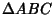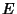## Triangle SquaringLetbe the Altitude of a Triangleand letbe its Midpoint. Thenandcan be squared by Rectangle Squaring. The general Polygon can be treated by drawing diagonals, squaring the constituent triangles, and then combining the squares together using the Pythagorean Theorem.

Dunham, W. Hippocrates' Quadrature of the Lune.'' Ch. 1 in Journey Through Genius: The Great Theorems of Mathematics. New York: Wiley, pp. 14-15, 1990.